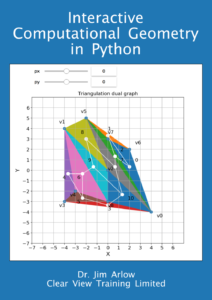# Interactive Computational Geometry in PythonAuthor: Jim Arlow, Clear View training Limited.

This book is an interactive introduction to some of the fundamental algorithms of computational geometry. It is supplied as a set of interactive Jupyter Notebooks.

Here are four sample chapters as non-interactive HTML pages. Because of limitations in Jupyter export to HTML, these chapters do not show the interactive demonstrations, they only show the text and a few figure. I am providing them so you can get some idea of what you will get in the book:

Introduction (open this to view the TOC for the whole book)

Chapter 1: Points and lines

Chapter 2: Polygonal chains in two dimensions

Chapter 3: Triangles and the relationship of points to lines